### 机器学习04：创建决策树

• 发布时间：2017年1月23日 16:38
• 作者：杨仕航

《机器学习实战》第2个算法是决策树。本来觉得该算法没什么，因为决策树原理很简单，实践也不复杂。仔细一看，原来它还包含自动构建较优的决策树，并绘制决策树。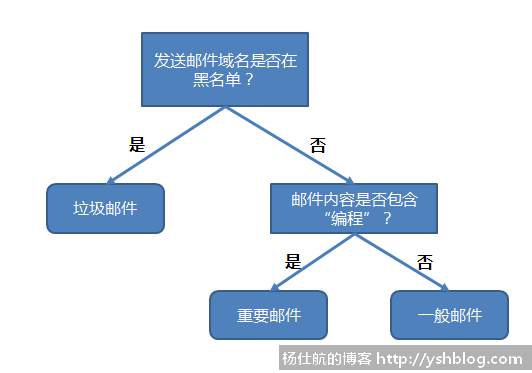1）收集数据：可以使用任何方法

2）准备数据：树构造算法只使用于标称型数据，因此数值型数据必须离散化

3）分析数据：可以使用任何方法。构造树完成之后，还需检查图形是否符合预期的效果

4）训练算法：构造树的数据结构

5）测试算法：计算错误率

6）使用算法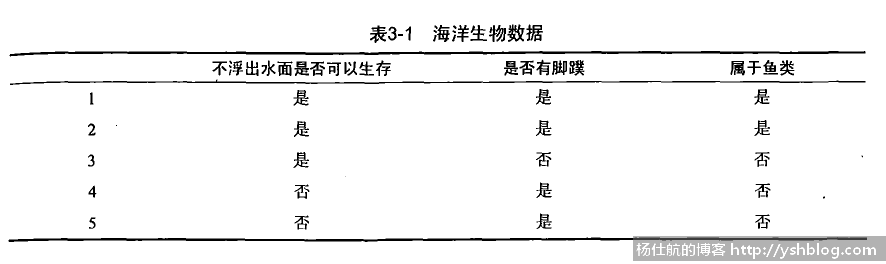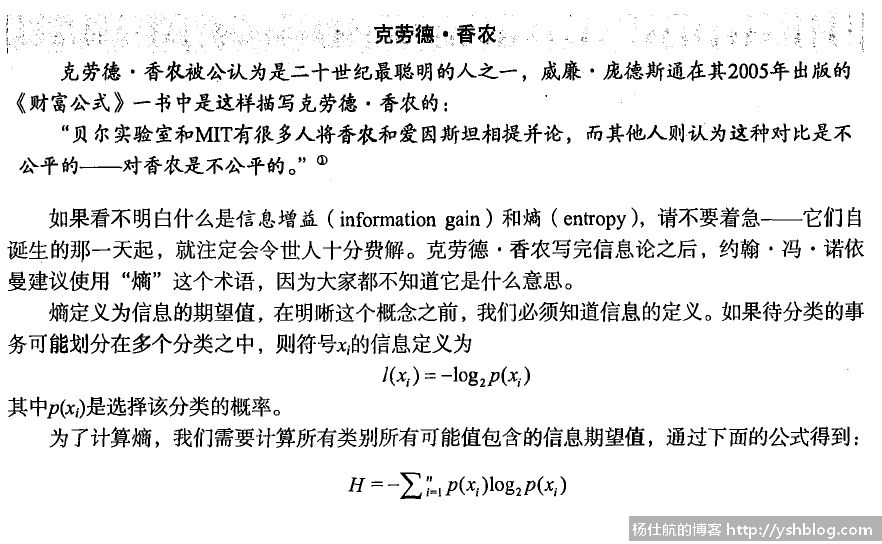```#coding:utf-8

def create_dataset():
"""创建测试数据集"""
dataset = [
[1,1,'yes'],
[1,1,'yes'],
[1,0,'no'],
[0,1,'no'],
[0,1,'no'],
]
labels = ['no surfacing', 'filppers']
return dataset, labels```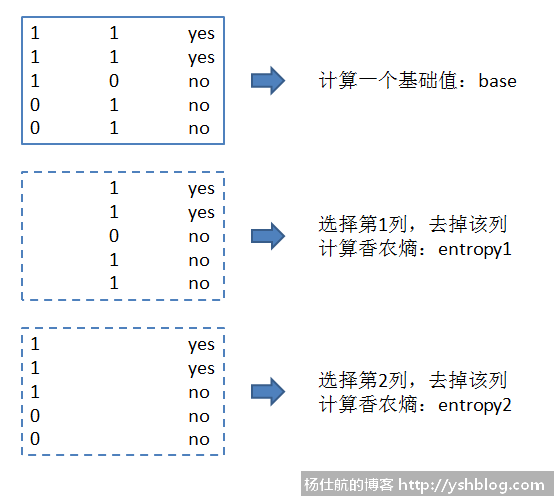```# -*- coding: utf-8 -*-
from math import log

#计算香农熵(dataset，每行最后一个值是类名)
def _calc_entropy(dataset):
num = len(dataset)
label_count = {}

for vect in dataset:
label = vect[-1]
label_count[label] = label_count.get(label, 0.) +1

entropy = 0.
for key,value in label_count.items():
prob = value/num #出现的概率
entropy -= prob * log(prob, 2)
return entropy

#划分数据集，剔除某一列的数据
def _filter_dataset(dataset, axis, value):
re_dataset = []
for vect in dataset:
if vect[axis] == value:
reduced_vect = vect[:axis] + vect[axis+1:] #移除元素
re_dataset.append(reduced_vect)
return re_dataset

#获取最好的划分方式
def best_split(dataset):
axis_num = len(dataset) - 1 #特征个数
base_entropy = _calc_entropy(dataset)
base_len = float(len(dataset))

best_info_gain = 0.
best_feature = -1

for i in range(axis_num):
feat_list = [x[i] for x in dataset] #特征值列表
feat_list = set(feat_list) #去重

new_entropy = 0.
for value in feat_list:
sub_dataset = _filter_dataset(dataset, i, value)
prob = len(sub_dataset)/base_len #比例
new_entropy += prob * _calc_entropy(sub_dataset)

#计算信息增益，并对比找到增益最多的特征
info_gain = base_entropy - new_entropy
if info_gain > best_info_gain:
best_info_gain = info_gain
best_feature = i
return best_feature```

```#返回最多数量的类别
def _majority_cnt(class_list):
class_count={}
for vote in class_list:
class_count[vote] = class_count.get(vote,0)+1
return max(class_count.iteritems(), key = lambda x:x)

#创建树
def create_tree(dataset, labels):
class_list = [x[-1] for x in dataset] #获取每条数据的类别
#若类别一样，就停止分类
if class_list.count(class_list) == len(class_list):
return class_list
#若无数据，则返回最多数量的类别？
if len(dataset) == 1:
return _majority_cnt(class_list)

#获取第1个分类特征
best_feat_axis = best_split(dataset)
best_feat_label = labels[best_feat_axis]

mytree = {best_feat_label:{}}
del(labels[best_feat_axis])

#获取剩下的特征顺序
feat_values = [x[best_feat_axis] for x in dataset]
unique_vals = set(feat_values)
for value in unique_vals:
#复制一个标签集
sub_labels = labels[:]
#递归处理
mytree[best_feat_label][value] = create_tree(_filter_dataset(dataset, best_feat_axis, value), sub_labels)
return mytree```

```if __name__ == '__main__':
dataset, labels = create_dataset()
mytree = create_tree(dataset,labels[:])

print(mytree)``````#利用决策树分类 classify(tree, labels, [1,0])
def classify(tree, labels, vect):
sub_key = tree.keys()
sub_dict = tree[sub_key]

index = labels.index(sub_key) #获取特征所在的位置
value = sub_dict[vect[index]] #获取树对应的值

#判断对应位置下的类别，若该类别是字典，则继续递归
if isinstance(value, dict):
class_label = classify(value, labels, vect)
else:
class_label = value
return class_label```

```#测试
test_vects = [[0,0],[0,1],[1,0],[1,1]]
for vect in test_vects:
result = classify(mytree, labels, vect)
print('%s:%s' % (vect, result))```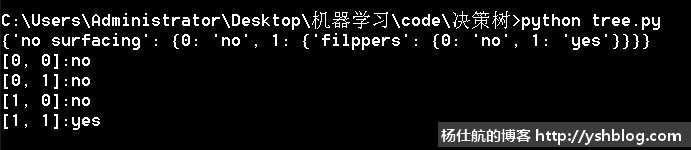#### 评论列表1271787323@qq.com

😕

2018-06-01 18:20